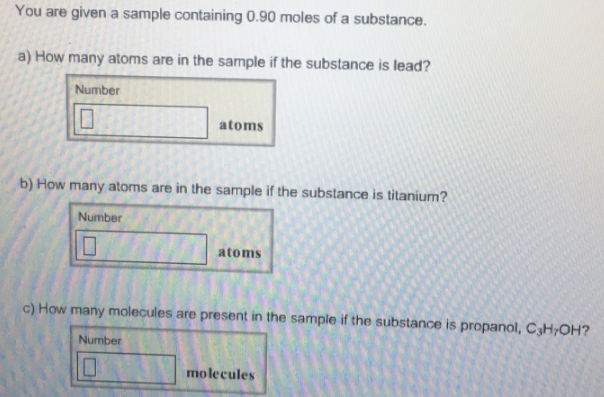# Problem: You are given a sample containing 0.90 moles of a substance. a) How many atoms are in the sample if the substance is lead? b) How many atoms are in the sample if the substance is titanium? c) How many molecules are present in the sample if the substance is propanol, C3H7OH?

###### FREE Expert Solution
99% (180 ratings)###### Problem Details

You are given a sample containing 0.90 moles of a substance.

a) How many atoms are in the sample if the substance is lead?

b) How many atoms are in the sample if the substance is titanium?

c) How many molecules are present in the sample if the substance is propanol, C3H7OH?